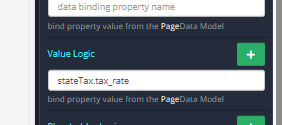# Populate a Input value from the value in the data table

I have a simple app where I have a list of dropdowns for Country, State and County

Currently i have the code set the property of the Input’s value to 6 where i have set the value logic field to stateTax. So every time the State is selected it puts a 6 in the value for that field

In my data table for each state it has a tax_rate column. How do i get the value to take the data table value rather than the static value of 6?

You can just expand on the data you are already loading into the dropdown so that you have the data in the same object as you are now using for the dropdown only.

Not sure if it is the best solution, but rather than loading data here directly into the select, I would store it in a Page data object first (and then do a map items from the page data object). Then I would use the On Change trigger for the select to get the currently selected value (objectId), find the matching objectId from your Page data object, and then update the tax_rate field with this value.

Some of the Backendless guys&gals can probably give you an easier solution than thisThank you Egil.

I got it working with the below code

and adding the Value logic to the Input field below